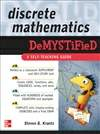# Discrete Mathematics Demystified: A Self-Teaching Guide

MULTIPLY your chances of understanding DISCRETE MATHEMATICS

If you're interested in learning the fundamentals of discrete mathematics but can't seem to get your brain to function, then here's your solution. Add this easy-to-follow guide to the equation and calculate how quickly you learn the essential concepts.

Written by award-winning math professor Steven Krantz, Discrete Mathematics Demystified explains this challenging topic in an effective and enlightening way. You will learn about logic, proofs, functions, matrices, sequences, series, and much more. Concise explanations, real-world examples, and worked equations make it easy to understand the material, and end-of-chapter exercises and a final exam help reinforce learning.

This fast and easy guide offers:

• Numerous figures to illustrate key concepts
• Sample problems with worked solutions
• Coverage of set theory, graph theory, and number theory
• Chapters on cryptography and Boolean algebra
• A time-saving approach to performing better on an exam or at work

Simple enough for a beginner, but challenging enough for an advanced student, Discrete Mathematics Demystified is your integral tool for mastering this complex subject.

# 下载地址

• 本书版权归原作者所有，本站暂无下载，请购买正版！
•